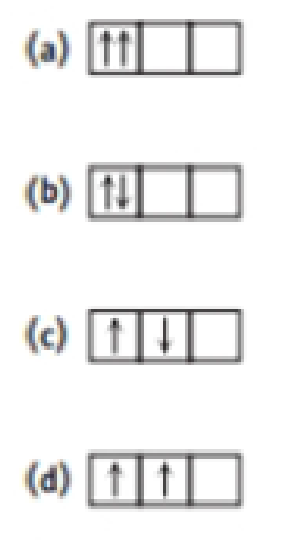Chapter 7, Problem 71SCQ

Chapter
Section
Textbook Problem

Compare the configurations below with two electrons located in p orbitals. Which would be the most stable (have the lowest energy)? Which would be the least stable? Explain your answers.Interpretation Introduction

Interpretation:

Compare the configurations and the most stable and least stable energy has to be predicted.

Concept Introduction:

Electronic configuration: The electronic configuration is the distribution of electrons of a given molecule or respective atoms in atomic or molecular orbitals. The important there rules for electronic configuration given below.

Aufbau principle: This rule states that ground state of an atom or ions electrons fill atomic orbitals of the lowest available energy levels before occupying higher levels. If consider the 1s shell is filled the 2s subshell is occupied.

Hund's Rule: The every orbital in a subshell is singly occupied with one electron before any one orbital is doubly occupied, and all electrons in singly occupied orbitals have the same spin.

Pauli exclusion rule: an atomic orbital may describe at most two electrons, each with opposite spin direction.

Explanation

Reason for correct options:

(d).[Morestabletwo electronsare parallel]pxpypz

The electronic configuration (d) is a more stable, because two electrons obeys Hund’s rule and  in (px and Py) shells. Than this configuration correctly marched for

Hence, the correct option is (d).

Reason for in-correct options:

(a).[NotobeyedforHund's Rule]pxpypz(b)

Still sussing out bartleby?

Check out a sample textbook solution.

See a sample solution

The Solution to Your Study Problems

Bartleby provides explanations to thousands of textbook problems written by our experts, many with advanced degrees!

Get Started